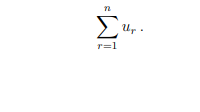# Sigma Notation Series Worksheets

What is Sigma Notation of a Series - Sigma notation is a method used to write out a long sum in a concise way. It is taken for convenience in order to write huge expressions in a simple and easy way. For example, we often wish to sum a number of terms such as 1 + 2 + 3 + 4 + 5 or 1 + 4 + 9 + 16 + 25 + 36 where there is an obvious pattern to the numbers involved. The first of these is the sum of the first five whole numbers, and the second is the sum of the first six square numbers. More generally, if we take a sequence of numbers u1, u2, u3, . . . , un then we can write the sum of these numbers as u1 + u2 + u3 + . . . + un. A shorter way of writing this is to let ur represent the general term of the sequence and putHere, the symbol Σ is the Greek capital letter Sigma corresponding to our letter 'S', and refers to the initial letter of the word 'Sum'.

• ### Basic Lesson

Guides students solving equations that involve an Sigma Notation Series. Demonstrates answer checking. Such a sequence summation is called a series and is designated by Sn where n represents the number of terms of the sequence being added.

• ### Intermediate Lesson

Demonstrates how to solve more difficult problems.

• ### Independent Practice 1

A really great activity for allowing students to understand the concept of Sigma Notation Series.

• ### Independent Practice 2

Students find the Sigma Notation Series in assorted problems. The answers can be found below.

• ### Homework Worksheet

Students are provided with problems to achieve the concepts of Sigma Notation Series.

• ### Skill Quiz

This tests the students ability to evaluate Sigma Notation Series.